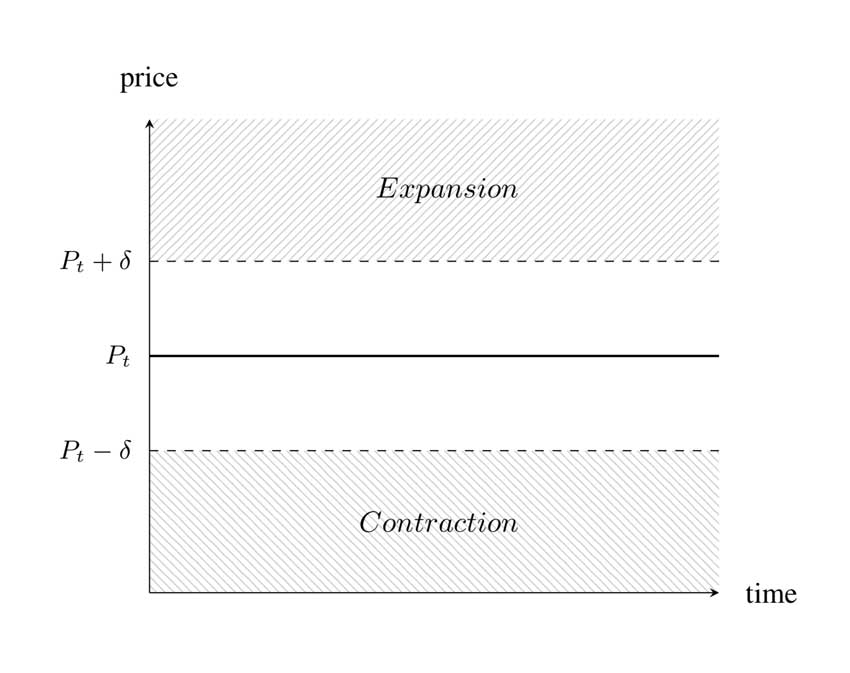## 1.3 Protocol Rules

To achieve price-supply equilibrium, the protocol expands and contracts supply in one of two ways. Given a price target, Pt and price threshold, δ:

• if the exchange rate between Amples and its target is > Pt + δ, the protocol responds by expanding to coin holders proportionally.
• if the exchange rate between Amples and its target is < Pt − δ, the protocol responds by contracting from coin holders proportionally.Above the threshold (Pt + δ) the protocol expands.
Below the threshold (Pt - δ) the protocol contracts.

The price target, Pt, is set to the purchasing power of one 2019 US dollar as represented by CPI. And the price threshold δ is set to 5%.

### Supply Smoothing

To avoid unnecessary overcorrection, the protocol grades supply changes as though they will distribute evenly over the course of 30 days. For example:

• if the exchange rate is 1.5 Amples : 1, the price difference can be offset by increasing each wallet’s balance by 50%.

Grading linearly over 30 days means in this case that the protocol will increase wallet quantities by +50% / 30 on the first day.
• if the exchange rate is 0.5 Amples : 1, this price difference can be offset by decreasing each wallet’s balance by −50%.

Grading linearly over 30 days in this case means that the protocol will update wallet quantities by −50% / 30 on day zero.

The supply change is recomputed and executed no more than once every 24 hours. This operation is stateless, meaning each day the protocol recomputes a supply target based on the latest price difference, and executes as though the change will occur evenly over the next 30 days without any memory of the previous day’s supply change.

Next: How Not to Trade Amples